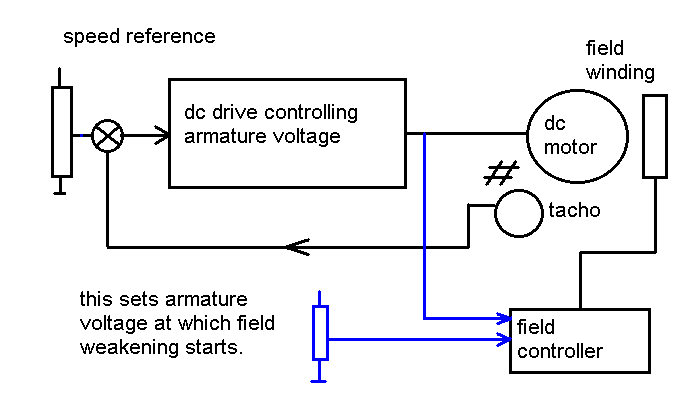Field weakening in a DC drive...

Speed of a DC motor is governed by two variables viz. Armature voltage and field voltage. Armature voltage and speed are directly proportional to each other. Field voltage and speed are inversely proportional to each other. i.e. if the field voltage is reduced keeping armature voltage constant, the speed of the motor goes up. On the other hand, if the field voltage is kept constant and armature voltage is increased, the speed goes up.

For getting speeds up to base speed of the motor ( designed speed which is achieved when armature voltage and field voltage are at their full rated values ), the armature voltage is varied from zero up to full rated voltage. For getting speed above the base speed, the armature voltage is kept constant and field is gradually reduced. This is represented in the sketch below.

##Electronically, using dc drives this is achieved as follows. Armature of the dc motor is connected to a normal dc drive which varies the speed of the motor from zero up to its rated base speed. This is achieved, for example, with a reference voltage of zero to 5 volts. At 5 volts reference level, the motor reaches its maximum level of armature voltage allowed. As the reference is further increased from 5 to 10 volts, another thyristor unit which feeds the field current, takes over the control and starts reducing the field slowly. This changeover of controls is smooth and automatic. Referring to the block diagram below makes it clear. Such controls are useful where the load on the motor ( kW ) does not increase proportionately at speeds abvoe certain speed. In this zone of speed, the higher speed of motor can be achieved through field weakening. In the zone where speed is increased by weakening of the field, the Horse Power of the motor remains constant and is therefore refered to as “Constant HP” operation. Below the point where field weakening just begins, the operation is called “Constant Torque”. These two names are slightly misleading. In the Constant HP zone, the horsepower actually developed is constant. However, in the Constant Torque zone, it is not the torque developed which is constant, but it is the Maximum Toruqe Available is constant.

 Block Diagram Ramp Circuit Speed Amplifier Current Amplifier Power Circuit Voltage feedback Vs Tacho feedback Current Limit Constant HP & constant Torque Field Weakening Motor runs at full speed Fuses Blow Hunting in speed Motor gives jerks Belts vibrate Speed drops on load Motor overheats Sparking on commutator DC drives and Power Factor Motor not able to drive the load DC to DC isolation Home About writer